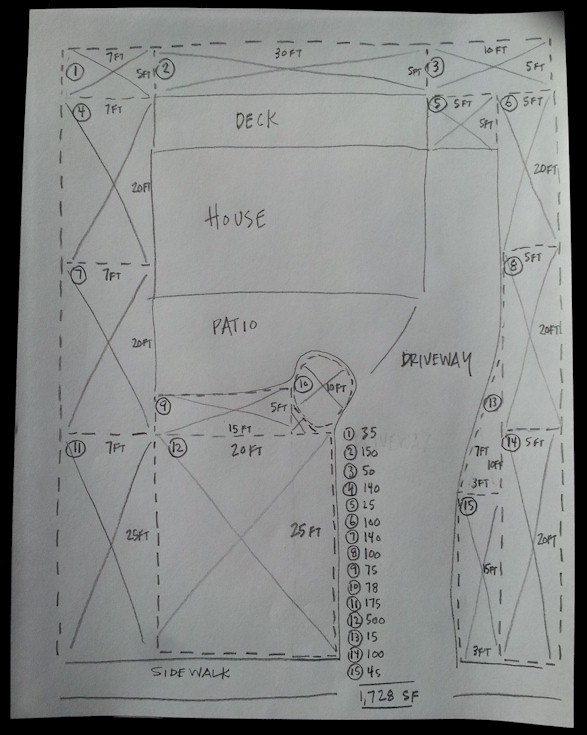| Sod Installation ■ Promote Your Business
 Sod Installation ProMatch Directory Cost Report

# How to Calculate the Amount of Sod Needed

Taking the time to accurately calculate how much sod you need will save you time and money.

How much time will it take? Depending on the shape and size of your yard it will only take you about 45 minutes to calculate how much sod you need.

What materials will I need? You’ll need a measuring tape, a pencil, graph paper and a calculator.

Measure carefully and always double check your measurements. It’s better to have too much sod than too little, so always round up or add an extra 5 or 10 percent to your total so end up with how much you need.

1. Sketch our your lawn into measurable squares, circles, and triangles.

Use your sketch pad to draw the shape of your entire lawn area. Then (like a puzzle) draw smaller (measurable) squares, rectangles, triangles, and circles on your sketch. If you need to, use fixed objects such as sidewalks, bushes, trees, sprinklers, etc. as benchmarks.

2. Measure each of your puzzle pieces.

You will need to measure the length, width, and height of each area identified on your sketch. For circles, you will need to estimate the radius (the line that extends from the center of the circle to the outside edge of the circle).

3. Calculate the area of each puzzle piece.

Here are a few formulas that you may need:

a) Area of a Square and Rectangle = Length x Width

b) Area of a Circle = Pi (3.14) x Radius (half the distance across the circle) x Radius (again)

c) Area of a Triangle = Base x Height ÷ 2

d) Area of a Trapezoid (four-sided non-square area) = Base 1 + Base 2 ÷ 2 x Height

e) Area of an Acres. 1 Acre = 43,560 square feet. Calculate: Acres x 43,560 = Area in Square Feet

4. Add the pieces together.

Once you have calculated the area of each puzzle piece, add them all together to get your total square foot requirement.